# Carboxylic Acids and Esters

The carboxylic acids are compounds containing the carboxyl functional group, $-COOH$. An example of a carboxylic acid is methanoic acid.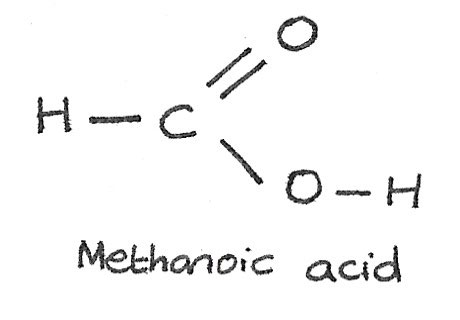#### Solubility

Short chain carboxylic acids are very soluble due to the polar $C=O$ and $O-H$ bonds which are able to form hydrogen bonds with the water molecules. As the number of carbon atoms increases, the solubility decreases as the length of the non-polar hydrocarbon chain increases.

## Acid Reactions

Carboxylic acids are weak acids compared with nitric, sulfuric and hydrochloric acids. They are acidic due to the ability to donate the hydrogen atom in the $-COOH$ group. Salts formed from carboxylic acids are called carboxylates.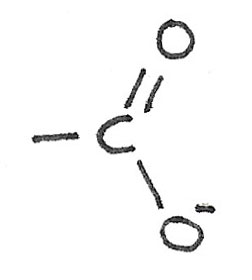### Reaction With Metals

When reacting with metals, a carboxylate salt and hydrogen gas is produced. Fizzing will be visible due to the release of hydrogen gas. In the reaction shown below, ethanoic acid reacts with potassium to form potassium ethanoate and hydrogen gas.

$$CH_{3}COOH + K \rightarrow CH_{3}COO^{-}K^{+} +\frac{1}{2}H_{2}$$

When forming these equations, it is important to consider the charge on the metal ion upon reacting with the acid. The magnesium ion has a positive charge of $\text{2+}$ while the carboxylate ion has a charge of $\text{1-}$. The equation must be balanced in order to balance these charges.

$$2CH_{3}COOH + Mg \rightarrow (CH_{3}COO^{-})_{2}Mg^{2+} + H_{2}$$

### Reaction with Aqueous Bases

Carboxylic acids react with aqueous bases such as metal hydroxides to form a salt and water.

$$CH_{3}COOH_{(l)} + NaOH_{(aq)} \rightarrow CH_{3}COO^{-}Na_{(aq)}^{+} + H_2O_{(l)}$$

### Reaction with Aqueous Carbonates

Carboxylic acids react with aqueous carbonates to form a salt, water and carbon dioxide. Fizzing will be visible due to the release of carbon dioxide.

$$2CH_{3}COOH_{(aq)} + K_{2}CO_{3(s)} \rightarrow 2CH_{3}COO^-K_{(aq)}^{+} + CO_{2(g)} + H_2O_{(l)}$$

## Esters

An ester compound is derived from a carboxylic acid, by replacing the hydrogen atom in the $-COOH$ group with a hydrocarbon chain.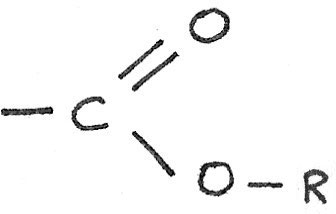Esters can be used as solvents, flavourings in foods and as perfumes.

### Esterification from carboxylic acids

Esters can be formed by reacting a carboxylic acid with an alcohol in the presence of a concentrated sulphuric acid catalyst. An example of an esterification reaction is shown below: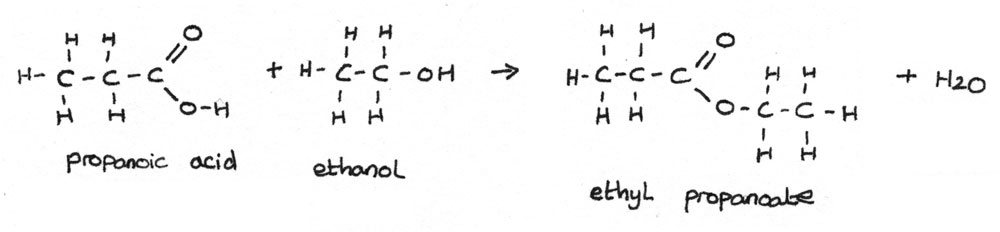$$CH_{3}CH_{2}COOH + CH_{3}CH_{2}OH \rightarrow CH_{3}CH_{2}COOCH_{2}CH_{3} + H_{2}O$$

When naming esters, the alkyl part at the start is derived from the alcohol. The second part which ends in -oate, is derived from the carboxylic acid group.

### Esters from acid anhydrides

Esters can also be formed by heating an acid anhydride with an alcohol. An acid anhydride is formed by joining together two carboxylic acids, for example: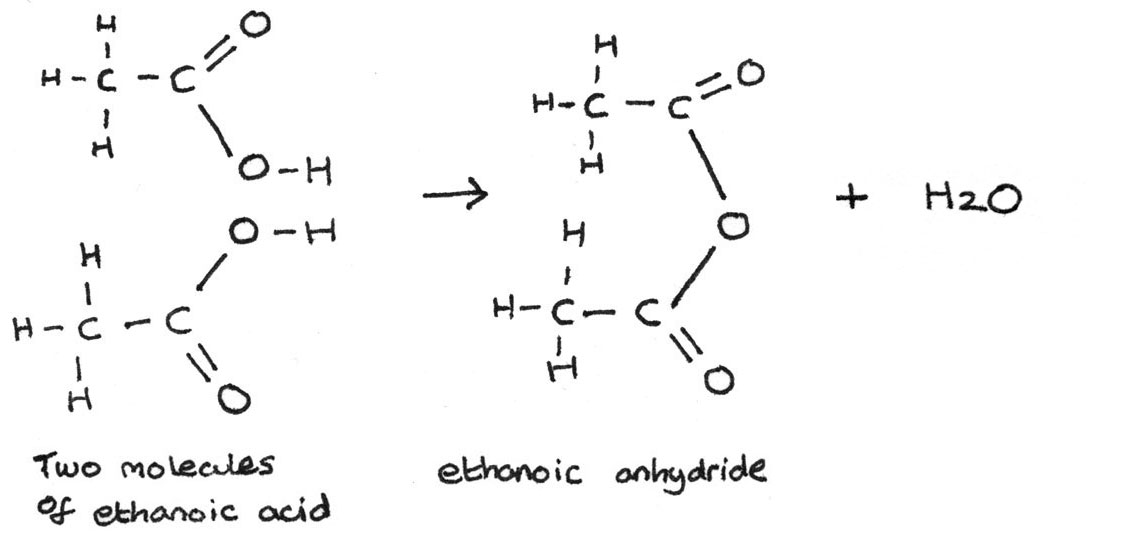The preparation of an ester from an acid anhydride and an alcohol produces a better yield than through esterification of carboxylic acids. The esterification reaction between ethanoic anhydride and methanol is shown below:

$$(CH_{3}CO)_{2}O + CH_{3}OH \rightarrow CH_{3}COOCH_{3} + CH_{3}COOH$$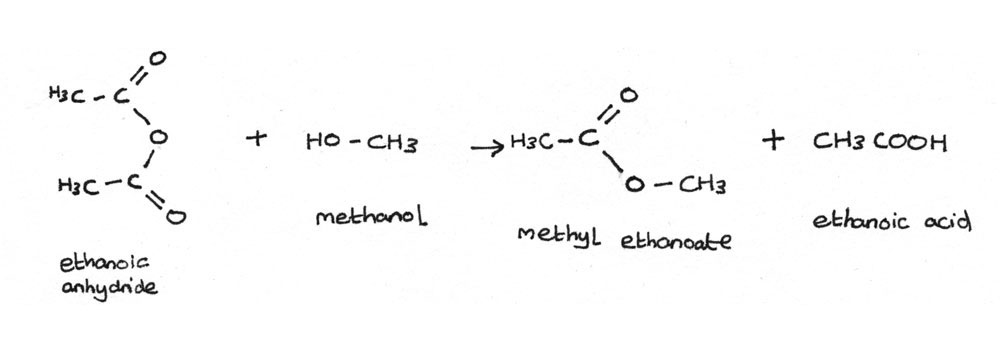## Hydrolysis of Esters

The hydrolysis of esters is the reverse reaction of esterification, where there is a chemical breakdown of the ester with water. It occurs with aqueous acids or aqueous alkalis.

### Acid Hydrolysis

In this reaction, the ester is heated under reflux with dilute hydrochloric acid. The ester reacts with the water, with the acid acting as a catalyst. A carboxylic acid and an alcohol is formed from the ester.

\eqalign{CH_{3}COOCH_{2}CH_{2}CH_{3} + H_{2}O &\rightleftharpoons CH_{3}COOH + CH_{3}CH_{2}CH_{2}OH \\ \text{propyl ethanoate} + \text{water} &\rightleftharpoons \text{ethanoic acid} + \text{propan-1-ol} }

### Alkaline Hydrolysis

Esters can also be hydrolysed under alkaline conditions, such as with aqueous sodium hydroxide or aqueous potassium hydroxide. This is a non-reversible reaction, which forms a carboxylate salt and an alcohol. This reaction can be called saponification and is used in the production of soaps.

$$CH_{3}CH_{2}COOCH_{2}CH_{3} + KOH \rightarrow CH_{3}CH_{2}COO^{-}K^{+} + CH_{3}CH_{2}OH \\ \text{ethyl propanoate} + \text{potassium hydroxide} \rightarrow \text{potassium propanoate} + \text{ethanol}$$